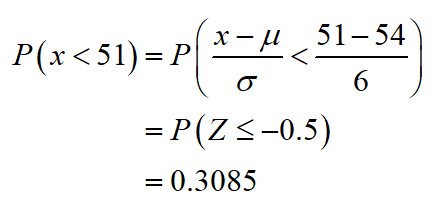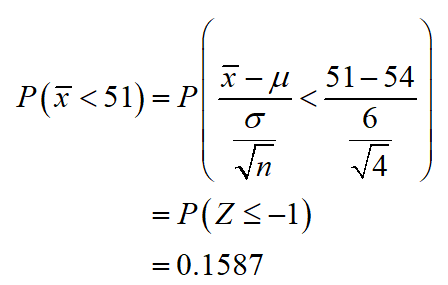# A normal distribution has a mean u = 54 and a standard deviation of sigma = 6.a) what is the probability of randomly selecting a score less than x = 51?b) what is the probability of selecting a sample  of n = 4 scores with a mean less than M = 51?c) what is the probability of selecting a sample of n = 36 scores with a mean less than M = 51?

Question
25 views

A normal distribution has a mean u = 54 and a standard deviation of sigma = 6.

a) what is the probability of randomly selecting a score less than x = 51?

b) what is the probability of selecting a sample  of n = 4 scores with a mean less than M = 51?

c) what is the probability of selecting a sample of n = 36 scores with a mean less than M = 51?

check_circle

Step 1

a)

The probability of randomly selecting a score less than x = 51 is,Thus, the probability of randomly selecting a score less than x = 51 is 0.3085.

Step 2

(b)

The probability of selecting a sample of n = 4 scores with a mean less than M = 51 is,Thus, the probability of selecting a sample of n=4 with a mean less than M=51 is 0.1587.s

...

### Want to see the full answer?

See Solution

#### Want to see this answer and more?

Solutions are written by subject experts who are available 24/7. Questions are typically answered within 1 hour.*

See Solution
*Response times may vary by subject and question.
Tagged in